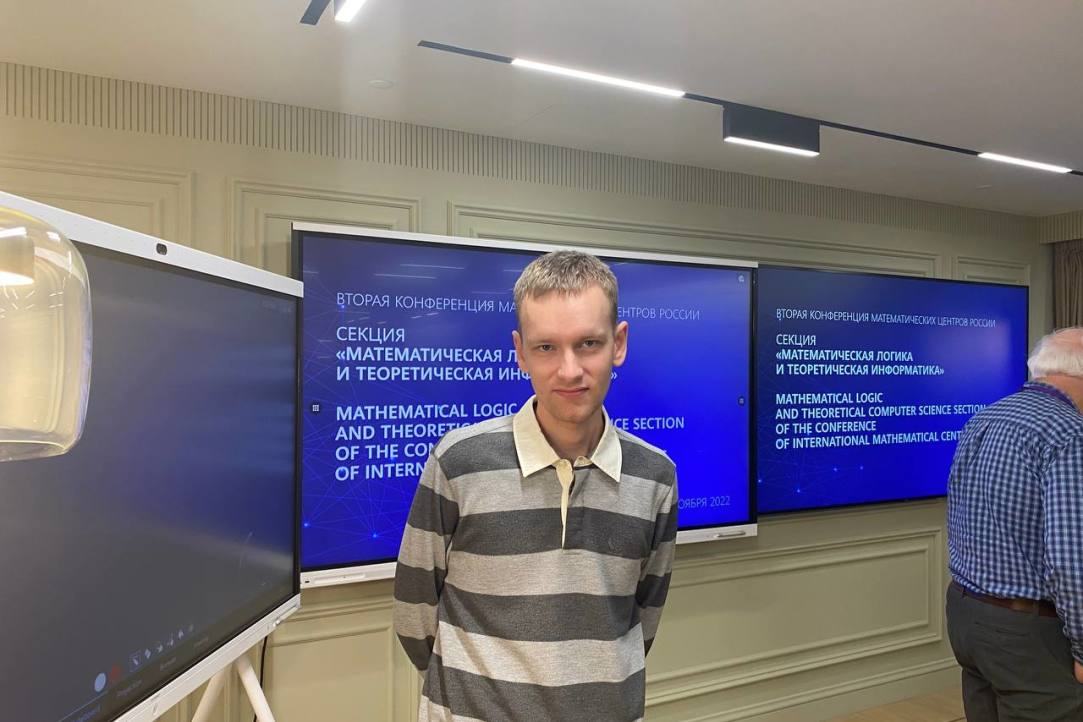• A
• A
• A
• АБВ
• АБВ
• АБВ
• А
• А
• А
• А
• А
Обычная версия сайта

# Александр Запрягаев выступил на второй конференции Математических центров России

С 7 по 11 ноября проходила вторая конференция Математических центров России (МГУ, МИАН). Александр Запрягаев выступил с докладом «Interpretations of Büchi arithmetics in themselves» на секции «Математическая логика и теоретическая информатика».Аннотация доклада

Büchi arithmetics BA_n, n ≥ 2, are extensions of Presburger arithmetic with an unary functional symbol V_n(x) denoting the largest power of n that divides x.  These theories were introduced by J. Büchi in order to describe the recognizability of sets of natural numbers by finite automata through definability in some arithmetical language. As shown by V. Bruyère, the definability of a set of natural numbers in BA_n is equivalent to its recognizability by a finite automaton receiving m-tuples of natural numbers in the form of m-tuples of their last, then penultimate, etc. digits of their n-ary expansion.

A. Visser has asked the following question: given an weak arithmetical theory T without ability to encode syntax but with full induction, does it hold that each interpretation (one-dimensional or multi-dimensional) of T into itself is isomorphic to the trivial one, and, if it is, is the isomorphism always expressible by a formula  of T? This question was previously answered positively for Presburger arithmetic PrA in the author’s joint work with F .Pakhomov.

We show that each interpretation of BA_n itself in its own standard model N and show that each such interpretation is isomorphic to the trivial one. Furthermore, we obtain this result already for the interpretations of Presburger Arithmetic in BA_n. The proof is based on the contradiction between a condition on automatic torsion-free abelian groups given by Braun and Strüngmann and the description of the order types of non-standard models of Büchi arithmetics. This gives a partial positive answer to the question of Visser.